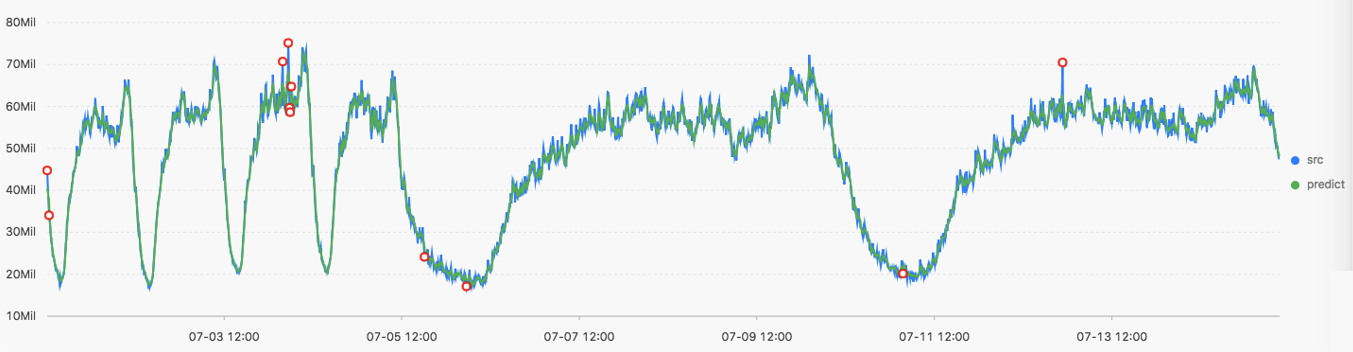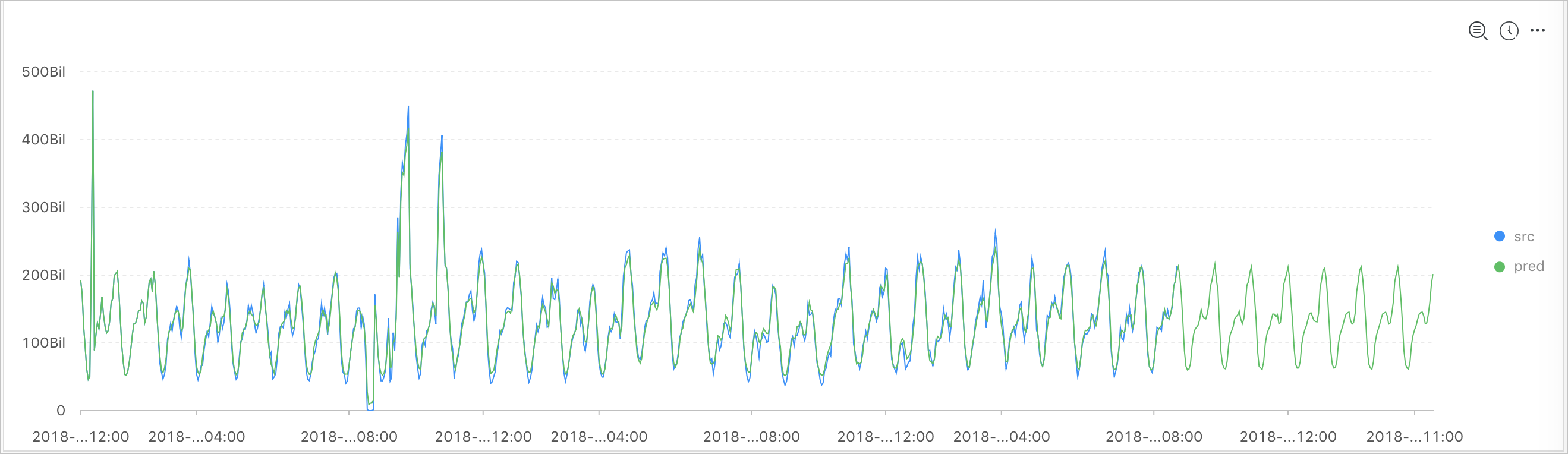To detect anomalies, you can use a prediction and anomaly detection function to predict a time series curve as well as identify the Ksigma and quantiles of the errors between a predicted curve and an actual curve.

## Function list

Function Description
ts_predicate_simple Uses default parameters to model time series data and performs simple time series prediction and anomaly detection.
ts_predicate_ar Uses an autoregressive model (AR) model to model time series data and performs simple time series prediction and anomaly detection.
ts_predicate_arma Uses an autoregressive moving average (ARMA) model to model time series data and performs simple time series prediction and anomaly detection.
ts_predicate_arima Uses an autoregressive integrated moving average (ARIMA) model to model time series data and performs simple time series prediction and anomaly detection.
ts_regression_predict Accurately predicts the trend for a periodic time series curve.

Scenario: This function can be used to predict metering data, network traffic, financial data, and different business data that follows certain rules.

ts_anomaly_filter Filters the anomalies detected during anomaly detection on multiple time series curves based on the custom anomaly mode. This function helps you quickly find abnormal curves.

## ts_predicate_simple

Function format:
``select ts_predicate_simple(x, y, nPred, isSmooth) ``
The following table lists the parameters of the function format.
Parameter Description Value
x The time sequence. Points in time are sorted in ascending order along the horizontal axis. Each point in time is a Unix timestamp. Unit: seconds.
y The sequence of numeric data corresponding to each specified point in time. N/A.
nPred The number of points for prediction. The value is of the long data type and must be equal to or greater than 1.
isSmooth Specifies whether to filter the raw data. The value is of the Boolean data type. The default value is true, which indicates that the raw data is to filter.
Example:
• The query statement is as follows:
``* | select ts_predicate_simple(stamp, value, 6) from (select __time__ - __time__ % 60 as stamp, avg(v) as value from log GROUP BY stamp order by stamp)``
• Output resultThe following table lists the display items.
Display item Description
Horizontal axis unixtime The Unix timestamp of the data. Unit: seconds.
Vertical axis src The raw data.
predict The data generated after the filtering operation is performed.
upper The upper limit of the confidence interval. The confidence level is 0.85, which cannot be modified.
lower The lower limit of the confidence interval. The confidence level is 0.85, which cannot be modified.
anomaly_prob The probability that the point is an anomaly. Valid values: [0, 1].

## ts_predicate_ar

Function format:
``select ts_predicate_ar(x, y, p, nPred, isSmooth) ``
The following table lists the parameters of the function format.
Parameter Description Value
x The time sequence. Points in time are sorted in ascending order along the horizontal axis. Each point in time is a Unix timestamp. Unit: seconds.
y The sequence of numeric data corresponding to each specified point in time. N/A.
p The order of the AR model. The value is of the long data type. Valid values: 2, 3, 4, 5, 6, 7, and 8.
nPred The number of points for prediction. The value is of the long data type. Valid values: [1, 5 × p].
isSmooth Specifies whether to filter the raw data. The value is of the Boolean data type. The default value is true, which indicates that the raw data is to filter.
An example of the query statement is as follows:
``* | select ts_predicate_ar(stamp, value, 3, 4) from (select __time__ - __time__ % 60 as stamp, avg(v) as value from log GROUP BY stamp order by stamp)``
Note The output result is similar to that of the ts_predicate_simple function. For more information, see the output result of the ts_predicate_simple function.

## ts_predicate_arma

Function format:
``select ts_predicate_arma(x, y, p, q, nPred, isSmooth) ``
The following table lists the parameters of the function format.
Parameter Description Value
x The time sequence. Points in time are sorted in ascending order along the horizontal axis. Each point in time is a Unix timestamp. Unit: seconds.
y The sequence of numeric data corresponding to each specified point in time. N/A.
p The order of the AR model. The value is of the long data type. Valid values: [2, 100].
q The order of the ARMA model. The value is of the long data type. Valid values: 2, 3, 4, 5, 6, 7, and 8.
nPred The number of points for prediction. The value is of the long data type. Valid values: [1, 5 × p].
isSmooth Specifies whether to filter the raw data. The value is of the Boolean data type. The default value is true, which indicates that the raw data is to filter.
An example of the query statement is as follows:
``* | select ts_predicate_arma(stamp, value, 3, 2, 4) from (select __time__ - __time__ % 60 as stamp, avg(v) as value from log GROUP BY stamp order by stamp) ``
Note The output result is similar to that of the ts_predicate_simple function. For more information, see the output result of the ts_predicate_simple function.

## ts_predicate_arima

Function format:
``select ts_predicate_arima(x, y, p, d, q, nPred, isSmooth) ``
The following table lists the parameters of the function format.
Parameter Description Value
x The time sequence. Points in time are sorted in ascending order along the horizontal axis. Each point in time is a Unix timestamp. Unit: seconds.
y The sequence of numeric data corresponding to each specified point in time. N/A.
p The order of the AR model. The value is of the long data type. Valid values: 2, 3, 4, 5, 6, 7, and 8.
d The order of the ARIMA model. The value is of the long data type. Valid values: [1, 3].
q The order of the ARMA model. The value is of the long data type. Valid values: 2, 3, 4, 5, 6, 7, and 8.
nPred The number of points for prediction. The value is of the long data type. Valid values: [1, 5 × p].
isSmooth Specifies whether to filter the raw data. The value is of the Boolean data type. The default value is true, which indicates that the raw data is to filter.
An example of the query statement is as follows:
``* | select ts_predicate_arima(stamp, value, 3, 1, 2, 4, 1, 'avg') from (select __time__ - __time__ % 60 as stamp, avg(v) as value from log GROUP BY stamp order by stamp)``
Note The output result is similar to that of the ts_predicate_simple function. For more information, see the output result of the ts_predicate_simple function.

## ts_regression_predict

Function format:
``select ts_regression_predict(x, y, nPred, algotype,processType)``
The following table lists the parameters of the function format.
Parameter Description Value
x The time sequence. Points in time are sorted in ascending order along the horizontal axis. Each point in time is a Unix timestamp. Unit: seconds.
y The sequence of numeric data corresponding to each specified point in time. N/A.
nPred The number of points for prediction. The value is of the long data type. Valid values: [1, 500].
algotype The algorithm type for prediction. Valid values:
• origin: uses the Gradient Boosted Regression Tree (GBRT) algorithm for prediction.
• forest: uses the GBRT algorithm for prediction based on the trend components decomposed by Seasonal and Trend decomposition using Loess (STL), and then uses the additive model to sum up the decomposed components and obtains the predicted data.
• linear: uses the Linear Regression algorithm for prediction based on the trend components decomposed by STL, and then uses the additive model to sum up the decomposed components and obtains the predicted data.
processType Specifies whether to preprocess the data. Valid values:
• 0: No additional data preprocessing is performed.
• 1: Abnormal data is removed before prediction.
Example:
• The query statement is as follows:
``* and h : nu2h05202.nu8 and m: NET |  select ts_regression_predict(stamp, value, 200, 'origin') from (select __time__ - __time__ % 60 as stamp, avg(v) as value from log GROUP  BY  stamp order by stamp)``
• Output resultThe following table lists the display items.
Display item Description
Horizontal axis unixtime The Unix timestamp of the data. Unit: seconds.
Vertical axis src The raw data.
predict The data generated after the filtering operation is performed.

## ts_anomaly_filter

Function format:
``select ts_anomaly_filter(lineName, ts, ds, preds, probs, nWatch, anomalyType)``
The following table lists the parameters of the function format.
Parameter Description Value
lineName The name of each curve. The value is of the varchar type. N/A
ts The time sequence of the curve, which indicates the time on the current curve. The parameter value is an array of points in time of the double data type sorted in ascending order. N/A
ds The actual value sequence of the curve. The parameter value is an array of data points of the double data type. This parameter value has the same length as the ts parameter value. N/A
preds The predicted value sequence of the curve. The parameter value is an array of data points of the double data type. This parameter value has the same length as the ts parameter value. N/A
probs The sequence of anomaly detection results of the curve. The parameter value is an array of data points of the double data type. This parameter value has the same length as the ts parameter value. N/A
nWatch The number of the recently observed actual values on the curve. The value is of the long data type. The value must be smaller than the number of points in time on the curve. N/A
anomalyType The type of the anomaly to filter. The value is of the long data type. Valid values:
• 0: all anomalies
• 1: positive anomalies
• -1: negative anomalies
Example:
• The query statement is as follows:
``````* | select res.name, res.ts, res.ds, res.preds, res.probs
from (
select ts_anomaly_filter(name, ts, ds, preds, probs, cast(5 as bigint), cast(1 as bigint)) as res
from (
select name, res as ts, res as ds, res as preds, res as uppers, res as lowers, res as probs
from (
select name, array_transpose(ts_predicate_ar(stamp, value, 10)) as res
from (
select name, stamp, value from log where name like '%asg-%') group by name)) );``````
• Output result
``````| name                     | ts                                                   | ds          | preds     | probs       |
| ------------------------ | ---------------------------------------------------- | ----------- | --------- | ----------- |
| asg-bp1hylzdi2wx7civ0ivk | [1.5513696E9, 1.5513732E9, 1.5513768E9, 1.5513804E9] | [1,2,3,NaN] | [1,2,3,4] | [0,0,1,NaN] |``````